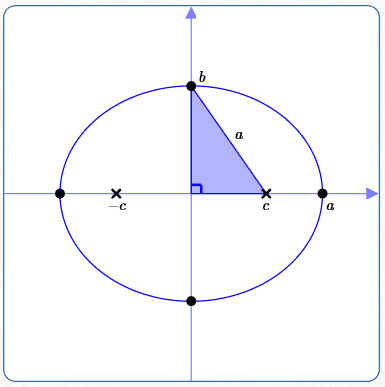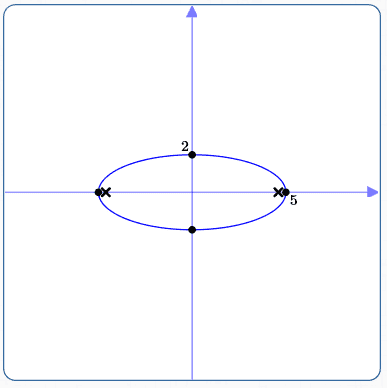﻿ Summary: Equations of Ellipses in Standard Form

# Summary: Equations of Ellipses in Standard Form

• PRACTICE (online exercises and printable worksheets)

## Summary: Equations of ellipses with centers at the origin and foci on the $x$-axis or $y$-axis

In both cases:
$\,a > b > 0\,$
The length of the major axis (which contains the foci) is $\,2a\,$.
The length of the minor axis is $\,2b\,$.
The foci are determined by solving the equation $\,c^2 = a^2 - b^2\,$ for $\,c\,$.

## Foci on the $x$-axisEquation of Ellipse: $$\frac{x^2}{\underset{\text{bigger}}{\underset{\uparrow}{a^2}}} + \frac{y^2}{b^2} = 1$$ When the foci are on the $\color{red}{x}$-axis,
the bigger number ($\,a^2 > b^2\,$) is beneath the $\,\color{red}{x}^2\,$.

Coordinates of foci: $\,(-c,0)\,$ and $\,(c,0)\,$

## Foci on the $y$-axisEquation of Ellipse: $$\frac{x^2}{b^2} + \frac{y^2}{\underset{\text{bigger}}{\underset{\uparrow}{a^2}}} = 1$$ When the foci are on the $\color{red}{y}$-axis,
the bigger number ($\,a^2 > b^2\,$) is beneath the $\,\color{red}{y}^2\,$.

Coordinates of foci: $\,(0,-c)\,$ and $\,(0,c)\,$

TIPS:
• The key to recognizing the equation of an ellipse with center at the origin and foci on either the $x$-axis or $y$-axis is this:
• It has only $\,x^2\,$, $\,y^2\,$, and constant terms.
• When the $\,x^2\,$ and $\,y^2\,$ terms are on the same side of the equation, then they must have the same sign.
• When the bigger number is beneath the $\,x^2\,$ term, then the foci are on the $\,x\,$-axis.
When the bigger number is beneath the $\,y^2\,$ term, then the foci are on the $\,y\,$-axis.
Look for the bigger denominator to tell you where the foci lie!
• Cut down on memorization—just use standard techniques to get the $x$ and $y$ intercepts.
For example, in the equation $\displaystyle\,\frac{x^2}{a^2} + \frac{y^2}{b^2} = 1\,$:
Let $\,y = 0\,$ to get the $x$-intercepts $\,a\,$ and $\,-a\,$:   $\displaystyle\,\frac{x^2}{a^2} = 1 \ \Rightarrow\ x^2 = a^2 \ \Rightarrow\ x = \pm a\,$.

Let $\,x = 0\,$ to get the $y$-intercepts $\,b\,$ and $\,-b\,$:   $\displaystyle\,\frac{y^2}{b^2} = 1 \ \Rightarrow\ y^2 = b^2 \ \Rightarrow\ y = \pm b\,$.
• To find the foci: $\,c^2\,$ is always the bigger denominator minus the smaller denominator. $$c^2 = \text{bigger denominator} - \text{smaller denominator}$$

## EXAMPLE: Graphing an Ellipse

Graph:   $100 - 4x^2 = 25y^2$

Initial thoughts:
There are only $\,x^2\,$, $\,y^2\,$ and constant terms.
When the $\,x^2\,$ and $\,y^2\,$ terms are on the same side of the equation, they have the same sign.
It's an ellipse with center at the origin and foci on either the $x$-axis or $y$-axis!

 Solution: $$\begin{gather} 100 - 4x^2 = 25y^2\cr\cr 4x^2 + 25y^2 = 100\cr\cr \frac{4x^2}{100} + \frac{25y^2}{100} = 1\cr\cr \frac{4x^2}{100}\cdot\frac{\frac 14}{\frac 14} + \frac{25y^2}{100}\cdot\frac{\frac 1{25}}{\frac 1{25}} = 1\cr\cr \frac{x^2}{25} + \frac{y^2}{4} = 1\cr\cr \end{gather}$$ $x$-intercepts (set $\,y = 0\,$):   $\,x = \pm 5\,$ $y$-intercepts (set $\,x = 0\,$):   $\,y = \pm 2\,$ Foci: The bigger number is under the $\,x^2\,$, so the foci are on the $x$-axis. $\,c^2 = 25 - 4 = 21\,$ $\,c = \pm\sqrt{21}$ $\,c\approx \pm 4.6\,$## EXAMPLE: Finding the Equation of an Ellipse

Find the equation of the following ellipse:

• center at the origin
• major axis along the $y$-axis, with length $\,8\,$
• minor axis has length $\,6\,$
Also, find the coordinates of the foci.

Solution:
• Since the major axis is along the $y$-axis, the form of the equation is $$\frac{x^2}{b^2} + \frac{y^2}{a^2} = 1$$
• $\,a\,$ is half the length of the major axis, which is $\,\frac{8}{2} = 4\,$
• $\,b\,$ is half the length of the minor axis, which is $\,\frac{6}{2} = 3\,$
• The equation is: $$\begin{gather} \frac{x^2}{3^2} + \frac{y^2}{4^2} = 1\cr\cr \frac{x^2}9 + \frac{y^2}{16} = 1 \end{gather}$$
• Foci:
$\,c^2 = a^2 - b^2 = 16 - 9 = 7\,$, so $\,c = \pm\sqrt{7}\,$
Coordinates of foci: $\,(0,-\sqrt{7})\,$ and $\,(0,\sqrt{7})\,$
Master the ideas from this section